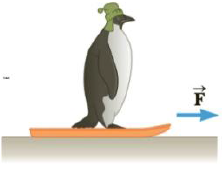# A sled weighing 60.0 N is pulled horizontally across snow so that the coefficient of kinetic friction between sled and snow is 0.100. A penguin weighing 70.0 N rides on the sled, as in Figure P4.86. If the coefficient of static friction between penguin and sled is 0.700, find the maximum horizontal force that can be exerted on the sled before the penguin begins to slide off. Figure P4.86### College Physics

11th Edition
Raymond A. Serway + 1 other
Publisher: Cengage Learning
ISBN: 9781305952300### College Physics

11th Edition
Raymond A. Serway + 1 other
Publisher: Cengage Learning
ISBN: 9781305952300

#### Solutions

Chapter
Section
Chapter 4, Problem 86AP
Textbook Problem

## A sled weighing 60.0 N is pulled horizontally across snow so that the coefficient of kinetic friction between sled and snow is 0.100. A penguin weighing 70.0 N rides on the sled, as in Figure P4.86. If the coefficient of static friction between penguin and sled is 0.700, find the maximum horizontal force that can be exerted on the sled before the penguin begins to slide off.Figure P4.86

Expert Solution
To determine
The maximum horizontal force.

### Explanation of Solution

Given info: The weight of the sled is 60.0 N. The weight of the penguin is 70.0 N. The co-efficient of kinetic friction is 0.100. The co-efficient of static friction is 0.700.

The formula for maximum acceleration is,

amax=μsg (I)

• μs is the co-efficient of static friction.
• g is the acceleration due to gravity.

The friction force opposes the horizontal force. Therefore,

Ff=(Wg)amax (II)

• F is the horizontal force.
• f is the friction force.
• W is the total weight.

Total weight is,

W=(60.0N)+(70

### Want to see the full answer?

Check out a sample textbook solution.See solution

### Want to see this answer and more?

Bartleby provides explanations to thousands of textbook problems written by our experts, many with advanced degrees!

See solution